Next: Problems Up: Notation and Conventions Previous: Pauli Matrices

# Useful Definitions

The following notation will be handy when dealing with spin-0 fields. For scaler functions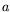and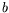,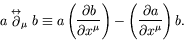(2.25)

The Dirac delta-function can be defined using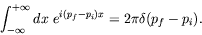(2.26)

A useful property of the Dirac delta-function is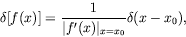(2.27)

where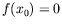. One particularly useful example of the above general relationship is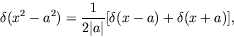(2.28)

whereis a constant.

The delta-function in three dimensions is often written as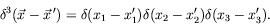(2.29)

Cauchy's integral formula is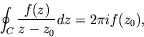(2.30)

where the direction of the contour of integration is clockwise. A counter-clockwise direction of integration results in an over all minus sign.

Integration by parts gives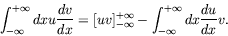(2.31)

The surface term''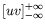can usually be neglected.Next: Problems Up: Notation and Conventions Previous: Pauli Matrices
Douglas M. Gingrich (gingrich@ ualberta.ca)
2004-03-18